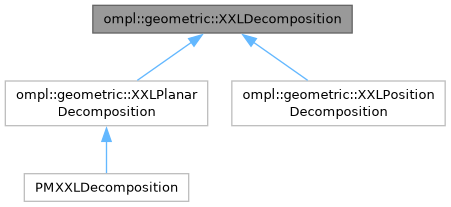ompl::geometric::XXLDecomposition Class Referenceabstract
Inheritance diagram for ompl::geometric::XXLDecomposition:[legend]

## Public Member Functions

XXLDecomposition ()
Constructor.

virtual int getNumRegions () const =0
Returns the number of regions in this XXLDecomposition.

virtual int getDimension () const =0
Return the dimension of this XXLDecomposition.

virtual int numLayers () const =0
Return the number of layers possible in this decomposition. Must be at least 1.

virtual int locateRegion (const base::State *s) const =0
Returns the index of the region containing a given State. Most often, this is obtained by first calling project(). Returns -1 if no region contains the State.

virtual int locateRegion (const std::vector< double > &coord) const =0
Return the region that this coordinate lies in. Returns -1 if this coord is out of bounds.

virtual void getNeighbors (int rid, std::vector< int > &neighbors) const =0
Stores the given region's neighbors into a given vector. These are adjacent neighbors.

virtual void getNeighborhood (int rid, std::vector< int > &neighborhood) const
Return a list of regions in the neighborhood of rid. This method is intended to retrieve all regions with in a 'radius'. This method should return a superset of getNeighbors (or equal). The neighborhood may or not be adjacent regions. Think of this as the 8-connected neighborhood of a grid, and getNeighbors as the 4-connected neighborhood of a grid.

virtual double distanceHeuristic (int r1, int r2) const =0
An admissible and consistent distance heuristic between two regions.

virtual bool sampleFromRegion (int r, base::State *s, const base::State *seed=nullptr) const =0
Sample a state s from region r in layer 0.

virtual bool sampleFromRegion (int r, base::State *s, const base::State *seed, int layer) const =0
Sample a state s from region r in the given layer.

virtual void project (const base::State *s, std::vector< double > &coord, int layer=0) const =0
Project the given State into the XXLDecomposition.

virtual void project (const base::State *s, std::vector< int > &layers) const =0
Project the state into the decomposition and retrieve the region for all valid layers.

virtual bool canSteer () const
Returns true if the method steerToRegion is available.

virtual bool steerToRegion (int, int, const ompl::base::State *, std::vector< ompl::base::State * > &) const

## Detailed Description

Definition at line 123 of file XXLDecomposition.h.

The documentation for this class was generated from the following file: